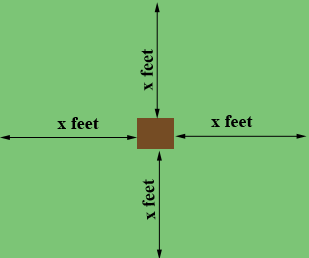SEARCH HOMEMath Central Quandaries & QueriesQuestion from Charlotte: If a house is 66x78 ft, how many feet out from the house around would be an acre to not include the houseHi Charlotte,

First you need to know how many square feet there are in an acre. Google can help with that. Type 1 acre in square feet into the Google search window.

Suppose the lot extends $x$ feet from the house in each direction.The house is 66 feet by 78 feet. What are the dimensions of the entire lot? What is the area of the entire lot? This area minus the area of the house is one acre, expressed in square feet. Solve for $x.$

PennyMath Central is supported by the University of Regina and The Pacific Institute for the Mathematical Sciences.